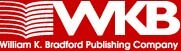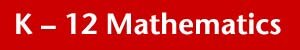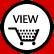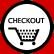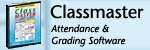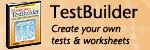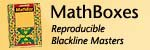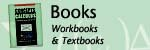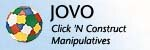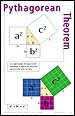Pythagorean TheormStatistics Formulas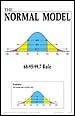The Normal Model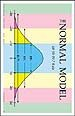The Normal Model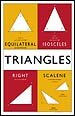TrianglesRoman Numerals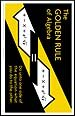The Golden Rule of Algebra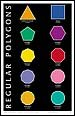Regular Polygons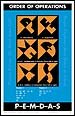Order of Operations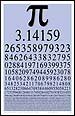Pi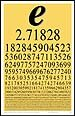Epsilon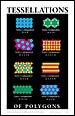Tessellations of Polygons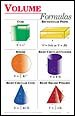Geometric FormulasMotivational Posters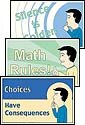Motivational Posters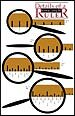Ruler Detail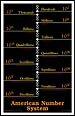American Number System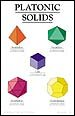Polyhedra PostersPolyhedra Posters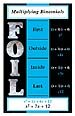Multiplying Binomials (FOIL)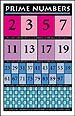Prime Numbers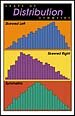Shape of Distribution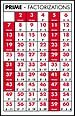Prime FactorizationTrigonometric Ratios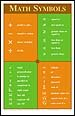Math Symbols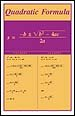Quadratic Formula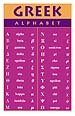Greek Alphabet